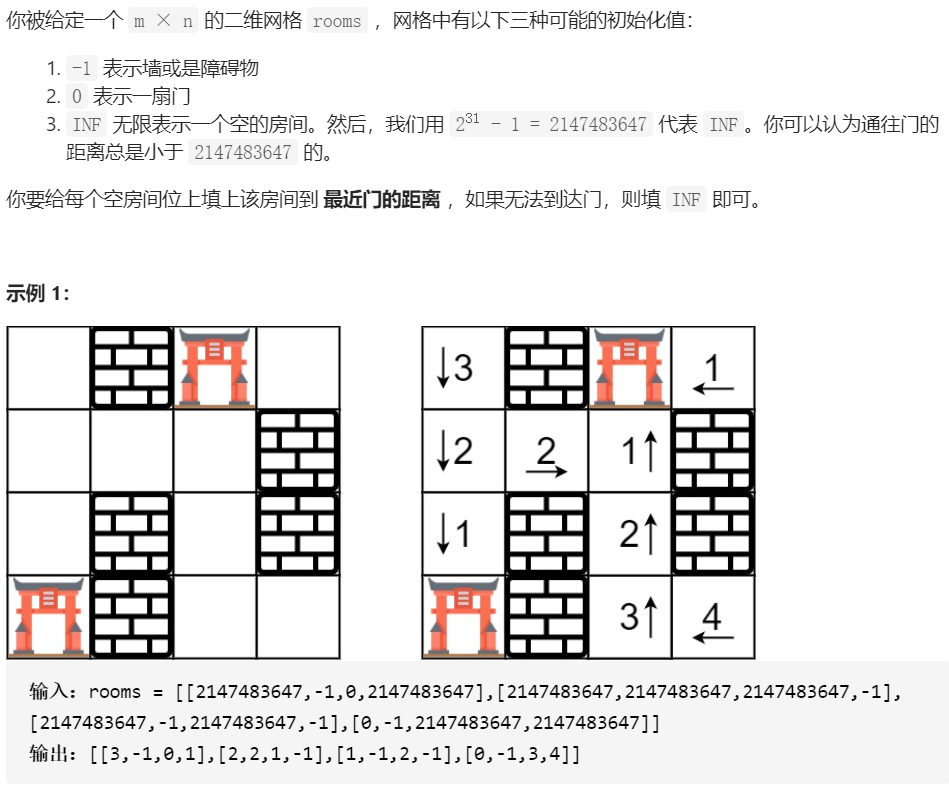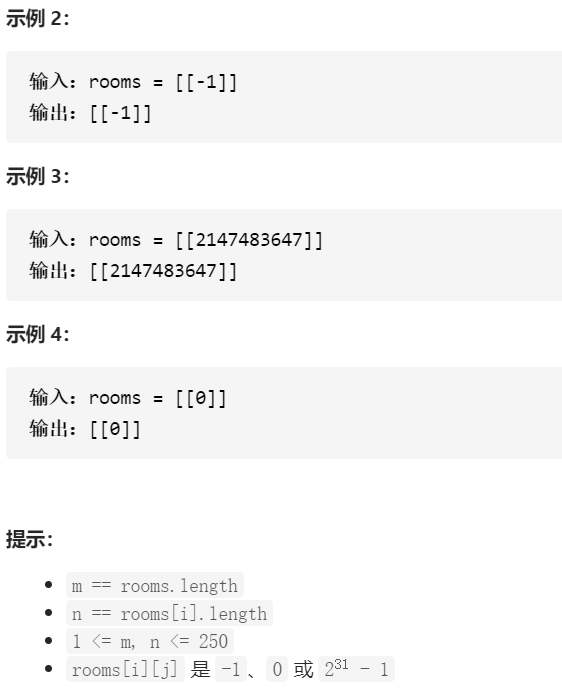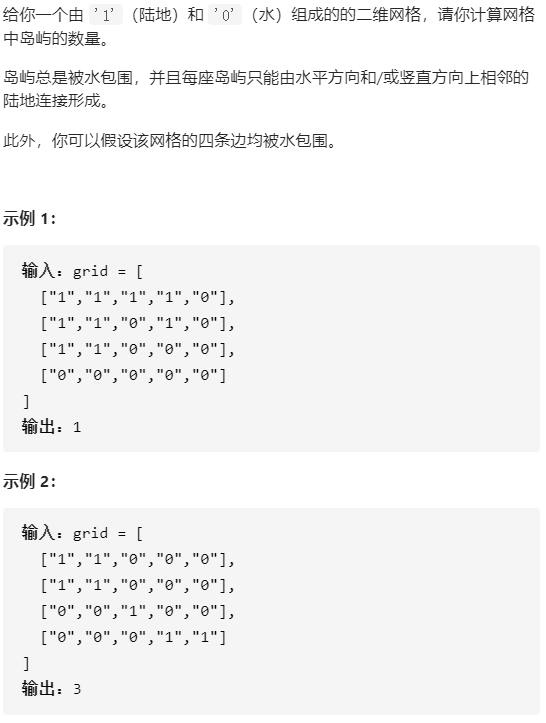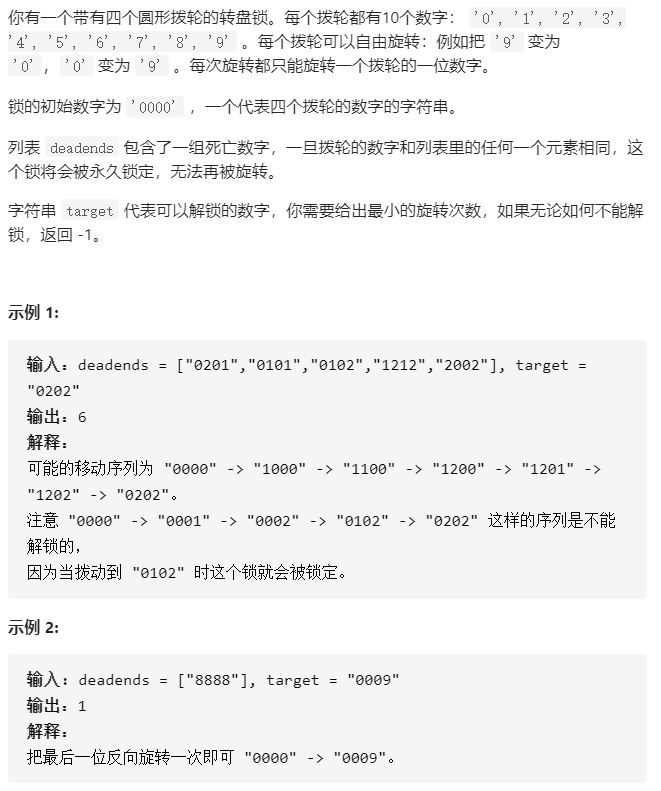• 数据结构课程设计——实现图的DFS和BFS算法C语言代码实现）
千次阅读
2021-02-03 18:00:51

### 一、实验目的及要求

分别构造四种类型图的邻接矩阵存储结构和邻接表存储结构。

1. 输出构造的图。
2. 分别实现图的DFS和BFS算法(存储结构任意)。
3. 在给定的连通网络上求解最小生成树（Prim算法和Kruscal算法任选）。
4. 在给定的有向带权图上实现求关键路径的算法。
5. 在带权的有向图上求解从某一原点出发到其余顶点的最短路径。
6. 以界面的形式展现出相关的选项。

### 二、实验代码

#include <stdio.h>
#include <stdlib.h>
#define INFINITY   10000
#define MAX_VERTEX_NUM    20//最大顶点数
typedef enum {DG, DN, UDG, UDN} GraphKind;
typedef struct ArcCell{//弧的信息结构
char      *info;
typedef struct {//图的结构
char    vex[MAX_VERTEX_NUM];//顶点向量
int   vexnum, arcnum;//图当前的顶点数与弧数
GraphKind  kind;
}MGraph;//图的邻接矩阵结构体定义
typedef struct ArcNode{
int adjvex;//邻接点域指示与顶点vi邻接的点数据结构 图的DFS算法 最小生成树算法
更多相关内容
• C语言BFS算法的实现C语言BFS算法的实现完整源码(定义，实现，main函数测试） C语言BFS算法的实现完整源码(定义，实现，main函数测试） #include <iostream> #include <vector> #include <queue> ...

# C语言BFS算法的实现完整源码(定义，实现，main函数测试）


#include <iostream>
#include <vector>
#include <queue>

namespace algo {

template <class dataType>                                 // Type of data vertex will hold
class
展开全文• 广度优先搜索（BFS）是一种遍历或搜索数据结构（如树或图）的算法。 如前所述，我们可以使用 BFS 在树中执行层序遍历。 我们也可以使用 BFS 遍历图。例如，我们可以使用 BFS 找到从起始结点到目标结点的路径，特别是...

## 编程总结

在刷题之前需要反复练习的编程技巧，尤其是手写各类数据结构实现，它们好比就是全真教的上乘武功。

本学习来自 leetcode，整理提炼.

广度优先搜索（BFS）是一种遍历或搜索数据结构（如树或图）的算法。
如前所述，我们可以使用 BFS 在树中执行层序遍历。
我们也可以使用 BFS 遍历图。例如，我们可以使用 BFS 找到从起始结点到目标结点的路径，特别是最短路径。
我们可以在更抽象的情景中使用 BFS 遍历所有可能的状态。在这种情况下，我们可以把状态看作是图中的结点，而以合法的过渡路径作为图中的边。

## 1. 解释队列和BFS的关系.

广度优先搜索（BFS）的一个常见应用是找出从根结点到目标结点的最短路径。

1. 结点的处理顺序是什么？
在第一轮中，我们处理根结点。在第二轮中，我们处理根结点旁边的结点；在第三轮中，我们处理距根结点两步的结点；等等等等。
与树的层序遍历类似，越是接近根结点的结点将越早地遍历。

如果在第 k 轮中将结点 X 添加到队列中，则根结点与 X 之间的最短路径的长度恰好是 k。也就是说，第一次找到目标结点时，你已经处于最短路径中。

1. 队列的入队和出队顺序是什么？
如上面的动画所示，我们首先将根结点排入队列。然后在每一轮中，我们逐个处理已经在队列中的结点，并将所有邻居添加到队列中。值得注意的是，新添加的节点不会立即遍历，而是在下一轮中处理。

结点的处理顺序与它们添加到队列的顺序是完全相同的顺序，即先进先出（FIFO）。这就是我们在 BFS 中使用队列的原因。

## 广度优先搜索 - 模板

之前，我们已经介绍了使用 BFS 的两个主要方案：遍历或找出最短路径。通常，这发生在树或图中。正如我们在章节描述中提到的，BFS 也可以用于更抽象的场景中。在本文中，我们将为你提供一个模板。然后，我们在本文后提供一些习题供你练习。在特定问题中执行 BFS 之前确定结点和边缘非常重要。通常，结点将是实际结点或是状态，而边缘将是实际边缘或可能的转换。

## 286. 墙与门// 反向思维，从门开始找
// 反向思维，从门开始找
typedef struct {
int x;
int y;
int step;
} Node;

void wallsAndGates(int **rooms, int roomsSize, int *roomsColSize)
{
if ((roomsSize == 0) || (*roomsColSize == 0)) {
return;
}
int row = roomsSize;
int col = roomsColSize;
int step = { {1,0},{-1,0},{0,1},{0,-1} }; // 下上右左
Node *queue = (Node *)malloc(sizeof(Node) * row * col);
int tail = 0;
int i, j;

// 找门，门节点都入队列.
for (i = 0; i < row; i++) {
for (j = 0; j < col; j++) {
if (rooms[i][j] == 0) {
queue[tail].x = i;
queue[tail].y = j;
queue[tail++].step = 0;
}
}
}
// 以门为起点开始BFS，利用该门节点遍历所有“岛”节点（这里借助了第200题岛的概念）
// 循环迭代直到队列为空
int curStep = queue[head++].step; // 门节点出队列
for (i = 0; i < 4; i++) {
int xNext = xx + step[i];
int yNext = yy + step[i];
if ((xNext >= 0) && (xNext < row) && (yNext >= 0) && (yNext < col) && (rooms[xNext][yNext] == INT_MAX)) {
rooms[xNext][yNext] = curStep + 1;
queue[tail].x = xNext;
queue[tail].y = yNext;
queue[tail++].step = curStep + 1;     // 将当前出队的元素相邻元素入队
}
}
}
}


## 200. 岛屿数量BFS : 思路是线性扫描整个二维网络，如果一个节点包含1，则以其为根节点启动BFS. 并将其设为2(FLAG_NUM)以标记访问过该节点，迭代地搜索队列中的每一个节点，直到队列为空 – 凡是能遍历到的都是一个岛上的. 继续此方法遍历二维网络其他点找其他岛，遍历完后就能找到所有“岛”的数量

#include <stdio.h>
#include <string.h>
#include <stdlib.h>
#include <math.h>

#define FLAG_NUM  2
typedef struct node {
int x;
int y;
} Node;

// BFS : 思路是线性扫描整个二维网络，如果一个节点包含1，则以其为根节点启动BFS.
// 并将其设为2(FLAG_NUM)以标记访问过该节点，迭代地搜索队列中的每一个节点，直到队列为空 -- 凡是能遍历到的都是一个岛上的.
// 继续此方法遍历二维网络其他点找其他岛，遍历完后就能找到所有“岛”的数量
void BFS(char **grid, int gridSize, int *gridColSize, int i, int j, int flag)
{
Node *queue = (Node *)malloc((gridSize*(gridColSize) + 1) * sizeof(Node));
Node tmp;
int step = {{1,0},{-1,0},{0,1},{0,-1}}; // 下上右左
int tail = 0;
tmp.x = i;
tmp.y = j;
queue[tail++] = tmp;                // 首元素入队列

// 循环迭代直到队列为空
int y = queue[head++].y;        // 当前点，出队列
for (int i = 0; i < 4; i++) {
int xNext = x + step[i];
int yNext = y + step[i]; // 遍历四个点
// 有符合条件的元素则加入队列
if (xNext >= 0 && xNext < gridSize && yNext >= 0 && yNext < gridColSize && grid[xNext][yNext] == '1') {
grid[xNext][yNext] = '0' + flag;
tmp.x = xNext;
tmp.y = yNext;
queue[tail++] = tmp;   // 入队列
}
}
}
}

int numIslands(char **grid, int gridSize, int *gridColSize)
{
int flag = FLAG_NUM;
int result = 0;

for (int i = 0; i < gridSize; i++) {
for (int j = 0; j < gridColSize; j++) {
if (grid[i][j] == '1') {
BFS(grid, gridSize, gridColSize, i, j, flag);
result++;
}
}
}

return result;
}

int main(void)
{
char **grid =  NULL;
int gridSize = 4;
int gridColSize = 5;

grid = (char **)malloc(sizeof(char *));
for (int i = 0; i < 4; i++) {
grid[i] = (char *)malloc(sizeof(char)*5);
}
grid = '1';
grid = '1';
grid = '1';
grid = '1';
grid = '0';
grid = '1';
grid = '1';
grid = '0';
grid = '1';
grid = '0';
grid = '1';
grid = '1';
grid = '0';
grid = '0';
grid = '0';
grid = '0';
grid = '0';
grid = '0';
grid = '0';
grid = '0';

numIslands(grid, gridSize, &gridColSize);

return 0;
}


DFS使用迭代，借助栈：

#define MAXSIZE   300
#define FLAG_NUM  2

typedef struct node
{
int x;
int y;
} Node;

void DFS(char **grid, int gridSize, int *gridColSize, int i, int j, int flag)
{
Node *stack = (Node *)malloc((gridSize*(gridColSize) + 1) * sizeof(Node));
Node tmp;
int top = -1;
tmp.x = i;
tmp.y = j;
stack[++top] = tmp; // 首元素入栈
int x;
int y;
int xNext;
int yNext;

while (top >= 0) {
x = stack[top].x;
y = stack[top].y;
xNext = x + 1;
yNext = y + 0;
if (xNext >= 0 && xNext < gridSize && yNext >= 0 && yNext < gridColSize && grid[xNext][yNext] == '1') {
grid[xNext][yNext] = '0' + flag;
tmp.x = xNext;
tmp.y = yNext;
stack[++top] = tmp;
continue; // 精髓在这里，直接跳出
}
xNext = x - 1;
yNext = y + 0;
if (xNext >= 0 && xNext < gridSize && yNext >= 0 && yNext < gridColSize && grid[xNext][yNext] == '1') {
grid[xNext][yNext] = '0' + flag;
tmp.x = xNext;
tmp.y = yNext;
stack[++top] = tmp;
continue;
}
xNext = x + 0;
yNext = y + 1;
if (xNext >= 0 && xNext < gridSize && yNext >= 0 && yNext < gridColSize && grid[xNext][yNext] == '1') {
grid[xNext][yNext] = '0' + flag;
tmp.x = xNext;
tmp.y = yNext;
stack[++top] = tmp;
continue;
}
xNext = x + 0;
yNext = y - 1;
if (xNext >= 0 && xNext < gridSize && yNext >= 0 && yNext < gridColSize && grid[xNext][yNext] == '1') {
grid[xNext][yNext] = '0' + flag;
tmp.x = xNext;
tmp.y = yNext;
stack[++top] = tmp;
continue;
}
top--;
}
}

int numIslands(char **grid, int gridSize, int *gridColSize){
int count   = 0;
int colSize = *gridColSize;

for (int i = 0; i < gridSize; i++) {
for (int j = 0; j < colSize; j++) {
if (grid[i][j] == '1') {
DFS(grid, gridSize, &colSize, i, j, 2);
count++;
}
}
}

return count;
}



DFS递归：

#define MAXSIZE 300
void DFS(char **grid, int gridSize, int colSize, int **visited, int x, int y)
{
if (x < 0 || x >= gridSize || y < 0 || y >= colSize) {
return ;
}

if (grid[x][y] == '1' && !visited[x][y]) {
visited[x][y] = 1;
DFS(grid, gridSize, colSize, visited, x + 1, y);
DFS(grid, gridSize, colSize, visited, x - 1, y);
DFS(grid, gridSize, colSize, visited, x, y + 1);
DFS(grid, gridSize, colSize, visited, x, y - 1);
}
}

int numIslands(char **grid, int gridSize, int *gridColSize){
int count   = 0;
int colSize = *gridColSize;
int **visited = (int **)malloc(sizeof(int *)*MAXSIZE);

for (int i = 0; i < MAXSIZE; i++) {
visited[i] = (int *)malloc(sizeof(int)*MAXSIZE);
memset(visited[i], 0, sizeof(int) * MAXSIZE);
};
// memset(visited[i], 0, sizeof(int) * MAXSIZE);
for (int i = 0; i < gridSize; i++) {
for (int j = 0; j < colSize; j++) {
if (grid[i][j] == '1' && !visited[i][j]) {
DFS(grid, gridSize, colSize, visited, i, j);
count++;
}
}
}

return count;
}


## 752.打开转盘锁#include <stdio.h>
#include <string.h>
#include <stdlib.h>
#include <math.h>

#define KEY_LENTH 4
#define QUEUE_LENTH 10000

typedef struct {
char key[KEY_LENTH + 1];
} Node;

// 构造循环队列
typedef struct {
Node *base;
int tail;
int MaxSize;
} Queue;

Queue *QueueCreate(int k)
{
Queue *obj = (Queue *)malloc(sizeof(Queue));
if (obj == NULL) {
return NULL;
}
obj->base = (Node *)malloc(sizeof(Node) * (k + 1));
memset(obj->base, 0, sizeof(Node) * (k + 1));
obj->tail = 0;
obj->MaxSize = k + 1;

return obj;
}

bool QueueIsEmpty(Queue  *obj)
{
return true;
}

return false;
}

bool QueueIsFull(Queue *obj)
{
return ((obj->tail + 1) == obj->head);
}

bool QueueEnQueue(Queue *obj, Node value)
{
if (QueueIsFull(obj) == true) {
return false;
}

obj->base[obj->tail] = value;
obj->tail = (obj->tail + 1) % obj->MaxSize;
return true;
}

bool QueueDeQueue(Queue *obj)
{
if (QueueIsEmpty(obj) == true) {
return false;
}

return true;
}

Node QueueFront(Queue *obj)
{
}

Node QueueTail(Queue *obj)
{
return obj->base[(obj->tail - 1)];
}

int QueueSize(Queue *obj)
{
return obj->tail + obj->MaxSize - obj->head;
}
}

void QueueFree(Queue *obj)
{
if (obj->base) {
free(obj->base);
}
obj->base = NULL;     // 先释放指针，并赋值NULL
obj->tail = 0;
free(obj);
}

//* -----------------------------  *//

Queue *gQueue;
int    gVisited[QUEUE_LENTH];

int Str2Int(char *s, int len)
{
int ret = 0;
for (int i = 0; i < len; i++) {
ret = ret * 10;
ret = ret + s[i] - '0';
}

return ret;
}

bool IsTarget(char *str, char *target)
{
return !strcmp(str, target);
}

{
for (int i = 0; i < deadendsSize; i++) {
gVisited[index] = 1;
}
}

{
for (int i = 0; i < deadendsSize; i++) {
if (IsTarget(str, deadends[i]) == true) {
return true;
}
}

return false;
}

{
/* 基于当前数字产生新的8位数字（各个位置前后旋转） */
for (int i = 0; i < KEY_LENTH; i++) {
for (int step = -1; step <= 1; step += 2) {
char nextCode[KEY_LENTH + 1];
memcpy(nextCode, str, 5);
nextCode[KEY_LENTH] = '\0';
nextCode[i] = (nextCode[i] - '0' + step + 10) % 10 + '0';
int index = atoi(nextCode);

continue;
}

Node enNode;
memcpy(enNode.key, nextCode, 5);
QueueEnQueue(gQueue, enNode);
gVisited[index] = 1;
}
}
}

{
int steps = 0;
char *str = (char *)"0000";

return -1;
}
gQueue = QueueCreate(QUEUE_LENTH);
memset(gVisited, 0, QUEUE_LENTH * sizeof(int));

// 将起点"0000"入队列
Node enNode;
memcpy(enNode.key, str, 5);
QueueEnQueue(gQueue, enNode);
gVisited = 1;

if (strcmp(str, target) == 0) {
return 0;
}

// 遍历队列
while (QueueIsEmpty(gQueue) == false) {
steps++;
int queueSize = QueueSize(gQueue);
for (int i = 0; i < queueSize; i++) { // 遍历已在队列中的节点
Node deNode;
deNode = QueueFront(gQueue);
QueueDeQueue(gQueue);
// 获取当前值的邻接值，判断是否在黑名单中，如不在，入队列
// 基于当前数字产生新的8位数字（各个位置前后旋转）
for (int i = 0; i < KEY_LENTH; i++) {
for (int step = -1; step <= 1; step += 2) {
char nextCode[KEY_LENTH + 1];
memcpy(nextCode, deNode.key, 5);
nextCode[KEY_LENTH] = '\0';
nextCode[i] = (nextCode[i] - '0' + step + 10) % 10 + '0'; // 这个手法的处理也是可以
int index = atoi(nextCode);
if (IsTarget(nextCode, target) == true) {
return steps;
}
if (gVisited[index] == 1) {
continue;
}
Node enNode;                     // 建立新的结点并入队列
memcpy(enNode.key, nextCode, 5);
QueueEnQueue(gQueue, enNode);
gVisited[index] = 1;
}
}
}
}
QueueFree(gQueue);
return -1;
}

int main(void)
{
char **deadends =(char **)malloc(sizeof(char *) * 5);
char *target = (char *)"0000";

for (int i = 0; i < 5; i++) {
}

return 0;
}

展开全文• 广度优先搜索算法BFS的相关代码，包括循环队列的代码

最近又有点学不进去了，不知道是不是天气热的缘故哈，没办法只好写一点算法来保持学习的路线不间断咯。 关于BFS和DFS，这是我们在面试的时候经常会遇到的两个基础算法，为什么说基础呢？因为它理解了之后才10行左右的代码，你说基础不基础？

一、 BFS

首先来用下面一颗的树来引入一下广度优先搜索的实现步骤：如上图所示，我们先用一棵树来引入广度优先搜索。为什么要用树呢？因为我觉得树来入门是最简单的，也是最容易理解的。

首先按照广度优先搜索的方法，我们就应该就是这样来搜索：

A->B->C->D->E->F

或者是：

A->C->B->F->D->E

我先来从结果分析上面的遍历结果，我发现广度优先搜索有这样的特点对：

先从根节点处查找，然后一层一层的往下查找。

怎么理解呢？首先查找A也就是第一层，然后再查找BC，也就是第二层，最后查找DEF 也就是第三层。

查找子层的时候，应该按照父层的顺序来查找子层。

怎么理解呢？首先查找A节点，然后查找A的子层B和C，当然我们在查找A子层的时候先来查找的B节点，那么在查找B的子节点的时候就要优先查找B的子节点。同理如果第二层里面先查找C也是一样。

广度优先搜索的搜索结果并不唯一。

通过上面的结果来分析，其实我们很容易发现广度优先算法有点队列的意味在里面。

怎么理解呢？来看下面一套图：首先新建一个队列，先去查找一下树的根结点，并且将根结点A放入队列中。移出节点A，并且把A的子节点加入到队列中。移出节点B，并且把B的子节点加入到队列中。

依次类推，就将所谓的最后的广度优先搜索的路线给画出来了：通过前面的探究，我们大概晓得了广度优先搜索的一个大致流程，也差不多知道其实现的规则有点类似于队列。此时可能有人会说了，你只是讲解了一个简单的树，而我们看到的BFS一般是用图来展示的。

针对上面的疑问，我们首先来画一个无向图。其实在我的理解里面：图和树最大的区别就是树有专门的起点，但是图却没有固定的起点。我们可以从任何一个点做起点来去搜索，例如：从A点出发，搜索结果是：

A->B->C->D->E->F

从E点出发：

E-> C->D->F->A->B

这样，我们其实就可以把之前树的那一套类似到图中，只不过图没有起点罢了，并且将树的子层换成了与指定节点相连的节点。这样类比之下也可以用队列来实现。具体的代码如下：

首先我们建立一张图：

let graph = {

'A':['B','C'],

'B':['A','C','D'],

'C':['A','D','E'],

'D':['B','C','E'],

'E':['C','D','F'],

'F':['E']

}

然后BFS的实现代码如下：

function bfs(graph,startPoint){

let queue = [];

let result = []

queue.push(startPoint);

result.push(startPoint);

while(queue.length>0){

let point = queue.shift();

let nodes = graph[point];

for(let node of nodes){

if(result.includes(node)) continue;

result.push(node);

stack.push(node);

}

}

return result

}

二、DFS

好了，前面谈到了广度优先搜索，那么什么是深度优先搜索呢？

首先我来用一套图集来辅助理解深度优先搜索的历程：首先选定起点为A(起点是任意的)，然后从A出发，搜索到B然后再从B出发，搜索到C再从C出发搜索到E再从E出发搜索到D，此时整张图都没有与D相连但是没被搜索到的节点了，此时该怎么办呢？如果搜索到没有可以搜索的节点，就应该回溯到最近存在相邻可以的节点处继续搜索。

总之，我对于深度优先搜索的理解就是：

访问顶点A

依次从A的未被访问的邻接点出发，对图进行深度优先遍历；直至图中和A有路径相通的顶点都被访问；

若此时图中尚有顶点未被访问，则从一个未被访问的顶点出发，重新进行深度优先遍历，直到图中所有顶点均被访问过为止。

这样看来，深度优先是不是有一点栈的意思在里面呢？怎么理解哦，我们再来看下面一套图。首先我们从A出发，将节点A移入栈，然后将A移出栈将A的子节点C，B移入栈内。然后将B移出栈，并将与B相连的D，C节点移入栈内然后将C移出栈，将与C相连的D，E节点移入栈内然后将E移出栈，将与E相连的F，D节点移入栈内然后将D移除栈，我们发现并没有可用的新节点了，就不再移入直接移出。移出F节点当我在移除新D节点的时候，发现D节点已经被移出过了。此时我们就将该节点丢弃，同样后面的节点也是一样。最后就完成了深度优先搜索。

通过上面的图级演示是不是很容易就能看出来深度优先搜索的实现原理呢？下面我们用代码的方式来去演示一下其原理吧：

function dfs(graph,startPoint){

let stack = [];

let result = []

stack.push(startPoint);

result.push(startPoint);

while(stack.length>0){

let point = stack.pop();

let nodes = graph[point];

for(let node of nodes){

if(result.includes(node)) continue;

result.push(node);

stack.push(node);

}

}

return result

}

说在最后

说了这么多，感觉午休的时间都所剩无几了，感觉自己还是没有把这部分的内容讲清楚。本来想额外写一点关于广度优先搜索的更深层次的用法的(作为很多图形结构的基础，其实应用还是比较广的)，但我还是需要睡的呀！反正中午看来是说不完了。关于还没写完的内容呢，我这里提及一下：

如果相邻节点之间的距离相同的情况下，我们想求广度优先搜索的最短路径怎么来求呢？

如果相邻节点之间的距离不同呢？我们应该如何来计算呢

如果明天中午有机会的话，我会细细讲解这一块的内容的，敬请期待哦！

最后最后提到一句，欢迎大家点赞，关注我的个人博客，我会源源不断的输出高质量文章的。

展开全文• 数据结构中重要的部分之一——图，这里主要完成一个无向无环图的建立，然后进行DFS BFS的遍历，输出结果，初学图和DFS BFS的小伙伴可以来看看噢
• 标准C的图的实现+BFS和DFS遍历+Dijkstra算法+Prim算法+Kruskal算法实现，纯手写！下载后如有疑问可以私信联系！全部手撸，一键运行，都封装成函数了，易读性很强
• BFS(基于C语言的简单实现) 把根结点放到队列的末尾 每次从队列的头部取出一个元素，查看这个元素所有的下一级元素，把它们放到队列的末尾。并把这个元素标记为它下一级元素的前驱 找到所要找的元素时...数据结构 广度搜索
• （二）最短路径BFS算法（无权图） //求顶点u到其他顶点的最短路径 void BFS_MIN_Distance(Graph G, int u){ //d[i]表示从u到i结点的最短路径 for(i=0; i<G.vexnum; i++){ d[i]=∞; //初始化路径长度最短路径问题 广度优先算法
• BFS，其英文全称是Breadth First Search。 广度优先搜索算法具有完全性。这意指无论图形的种类如何，只要目标存在，则BFS一定会找到。然而，若目标不存在，且图为无限大，则BFS将不收敛（不会结束）。 广度优先搜索...数据结构
• 由于看论文需要，最近自学数据结构到图这一章，用C语言写了一个使用邻接表建立图的程序，最后用BFS算法对顶点进行遍历，DFS较为简单，就不写了。 #include<stdio.h> #include<stdlib.h> #define ...数据结构 队列
• 0x02、使用C语言实现广度优先搜索 广度优先搜索使用队列实现，基本写法如下： void BFS(int s){ queue q; q.push(s); while(!q.empty()){ 取队首; 访问队首; 弹出队首; 队首元素下一层级的元素全部入队; } } ...
• 广度优先法(BFS)算法C/C++代码，要说明和图解！谢谢！#include #define MAX 10 int front=-1,rear=-1; struct node { int value; struct. return queue[front]; } void bfs(int current) //广度优先 { link tempnode...
• 目录 一，bfs（广度优先搜索）的定义 二,bfs(广度优先搜索)的应用 三，题型训练 1，奇怪的电梯 ...一，bfs（广度优先搜索）的定义 ...BFS 全称是Breadth ...这样做的结果是，BFS 算法找到的路径是从起点开始的最短...
• main.c #include "DBFS.h" int main() { Graph G[N][N]; Visit visit[N]; InitGraph(G,visit);... printf("BFS:\n"); InitGraph(G,visit); BFS(G,visit,1); } DBFS.c #include"DBFS.h" #include"Queue.h开发语言 数据结构
• ## BFS算法（详细C）

万次阅读 多人点赞 2017-02-24 09:43:35
最近学了图的广度和深度优先遍历，但是广度比深度要麻烦一些，用到了队列，我就完全的按自己的思路写了一段很长的代码，看了一些大神写的，但其实可以比较简单，其实图也有多种表示方式，所以各个代码会有不同，下面...数据结构 遍历
• C语言实现BFS算法queue结构体,Graph结构体,node结构体BFS算法完整源码（定义，实现，main函数测试） queue结构体,Graph结构体,node结构体 struct queue { int items[SIZE]; int front; int rear; }; struct Graph...
• } } void BFS(Lgraph *p,Queue *q,int source) //要完成BFS算法，需要队列中的创建 增加删除操作 { Anode *current; int i,u; int visited[MaxVex]; for(i=0;ivnum;i++) { visited[i]=0; //设置一个标志数组用来...
• 在图1，3*3的方格棋盘上，摆放着1到8这八个数码，有1个方格是空。 如图1所示，要求对空格执行空格左移、空格右移、空格上移和空格下移这四个操作使得棋盘从初始状态（图1左）到目标状态（图1右）。...
• ## C语言 -- BFS

千次阅读 2020-04-30 20:18:39
BFS（广度优先搜索） 常用来解决最短路径问题。第一次遍历到目的节点时，所经过的路径是最短路径。 几个要点： 1、只能用来求解无权图的最短路径问题 2、队列：用来存储每一层遍历得到的节点 3、标记：对于遍历...
• 规格为A4纸或A3纸折叠 佛山科学技术学院用四号宋体 实 验 报 告用小二号黑体 课程名称 数据结构实验 实验项目 实现DFS和BFS算法 专业班级 姓 名 学 号 指导教师 成 绩 日 期 用小四号宋体 一目的与要求 1通过本实验...
• 算法时间复杂度为O(|V|^2) 4 注意对每个连通分量调用了一次BFS，不然可能存在只访问一个连通分量的问题 BFS算法一般思路 下图对应无向图的遍历序列为abcdefgh： 对应无向图的遍历序列为abcdefgh 具体代码（邻接矩阵...
• void BFS (char** grid, int gridSize, int colSize, short (*viste)[MAXSIZE], int x, int y) { Node_s que[MAXSIZE * MAXSIZE]; memset(que, 0, sizeof(Node_s) * MAXSIZE * MAXSIZE); int right = 0; int left =...
• 前面介绍广度优先算法的时候提及了多次走迷宫，我们就真正的走一次迷宫试试！要求如下：输入给出迷宫矩阵的行数和列数，并给出迷宫(使用点 (.) 表示路，使用星 (*) 表示障碍物，使用S表示起点，T表示终点)例如：5 5....
• 一、图的广度优先遍历BFS （一）树 vs 图 ⼴度优先遍历（Breadth-First-Search, BFS）要点： ①、找到与⼀个顶点相邻的所有顶点 ②、标记哪些顶点被访问过 ③、需要⼀个辅助队列 FirstNeighbor(G，x)：求图G中顶点...
• (迪杰斯特拉算法描述) /* 算法思路： 1.逐步地发展最短路径树，直至它覆盖所有顶点。 2.构造一个循环，每次循环都增加一个顶点到最短路径树上。 3.从所有与树邻接的顶点中，选择离源点最近的。 4.对每个顶点，都用一...c++
• 对于8数码问题，有BFS算法和DFS算法两种方法，对于DFS来说，要优先设置搜索的深度，别的不多说，直接上代码。BFS的实现是用C语言的队列的知识，结点是一个结构体。 DFS的实现是用C语言的栈的知识点，结点时一个...数据结构...